# Newton's Laws of Motion Review

## Navigate to:

Review Session Home - Topic Listing

Newton's Laws - Home  ||  Printable Version  ||  Questions with Links

Answers to Questions:  All  ||  #1-7  ||  #8-36  ||  #37-46  ||  #47-60

## Part A: Mass, Inertia, Weight, and Newton's First Law of Motion

1. Which of the following statements are true of inertia? List all that apply.

1. Inertia is a force.
2. Inertia is a force which keeps stationary objects at rest and moving objects in motion at constant velocity.
3. Inertia is a force which brings all objects to a rest position.
4. All objects have inertia.
5. A more massive object has more inertia than a less massive object.
6. Fast-moving objects have more inertia than slow-moving objects.
7. An object would not have any inertia in a gravity-free environment (if there is such a place).
8. Inertia is the tendency of all objects to resist motion and ultimately stop.
9. In a gravity-free environment (should there be one), a person with a lot of inertia would have the same ability to make a turn as a person with a small amount of inertia.
 Answer: DE a. False - Inertia is not a force. b. False - Inertia is NOT a force. c. False - Inertia is NOT a force. Inertia is simply the tendency of an objects to resist a change in whatever state of motion that it currently has. Put another way, inertia is the tendency of an object to "keep on doing what it is doing." Mass is a measure of an object's inertia. The more mass which an object has, the more that it sluggish towards change. d. True - Bet money on this one. Any object with mass has inertia. (Any object without mass is not an object, but something else like a wave.) e. True - Mass is a measure of an object's inertia. Objects with greater mass have a greater inertia; objects with less mass have less inertia. f. False - The speed of an object has no impact upon the amount of inertia that it has. Inertia has to do with mass alone. g. False - Inertia (or mass) has nothing to do with gravity or lack of gravity. In a location where g is close to 0 m/s/s, an object loses its weight. Yet it still maintains the same amount of inertia as usual. It still has the same tendency to resist changes in its state of motion. h. False - Inertia is NOT the tendency to resist motion, but rather to resist changes in the state of motion. For instance, its the tendency of a moving object to keep moving at a constant velocity (or a stationary object to resist changes from its state of rest). i. False - Once more (refer to g), inertia is unaffected by alterations in the gravitational environment. An alteration in the g value effects the weight of an object but not the mass or inertia of the object.

 Useful Web Links Inertia and Mass

[ #1 | #2 | #3 | #4 | #5 | #6 | #7 | #8 | #9 | #10 | #11 | #12 | #13 | #14 | #15 | #16 | #17 | #18 | #19 | #20 | #21 | #22 | #23 | #24 | #25 | #26 | #27 | #28 | #29 | #30 | #31 | #32 | #33 | #34 | #35 | #36 | #37 | #38 | #39 | #40 | #41 | #42 | #43 | #44 | #45 | #46 | #47 | #48 | #49 | #50 | #51 | #52 | #53 | #54 | #55 | #56 | #57 | #58 | #59 | #60 ]

2. Which of the following statements are true of the quantity mass? List all that apply.

1. The mass of an object is dependent upon the value of the acceleration of gravity.
2. The standard metric unit of mass is the kilogram.
3. Mass depends on how much stuff is present in an object.
4. The mass of an object is variable and dependent upon its location.
5. An object would have more mass on Mount Everest than the same object in the middle of Lake Michigan.
6. People in Weight Watcher's are really concerned about their mass (they're mass watchers).
7. The mass of an object can be measured in pounds.
8. If all other variables are equal, then an object with a greater mass would have a more difficult time accelerating.
9. If all other variables are equal, then it would require less exerted force to stop a less massive object than to stop a more massive object.
10. The mass of an object is mathematically related to the weight of the object.

a. False - Mass is independent of the gravitational environment that an object is in and dependent solely upon the number of atoms in the object and the type of atoms (Carbon: ~12 g/mol; Hydrogen: ~1 g/mol ; Oxygen: ~16 g/mol). Because of this, mass is said to be invariable (unless of course, an object loses some of its atoms) - a constant quantity which is independent of the acceleration of gravity and therefore independent of location. (Weight on the other hand depends upon the gravitational environment.)

b. True - Know this one. Kilograms is for mass and Newtons is for force.

c. True - This is kind of a simple definition of mass but it does do the job (provided stuff means atoms or material).

d. False - See explanation to #2d.

e. False - An object has the same mass on Mount Everest as it does at sea level (or near sea level); only the weight of the object would be slightly different in these two locations.

f. True - Weight Watcher's participants only use a measurement of their weight as a reflection of how many atoms of flesh that they have burned from their bodies. Their real interest is in losing mass for reasons related to health, appearance, etc.

g. False - Pounds is a unit of force commonly used in the British system of measurement. It is not a metric unit and it is not a unit of mass. Kilogram is the standard metric unit of mass and slug is the British unit.

h. True - Weight and force of gravity are synonymous terms. You should quickly become comfortable with the terms mass, weight and force of gravity; it will save you many headaches as we continue through the course.

i. True - A less massive object has less inertia and as such would offer less resistance to changes in their velocity. For this reason, a less massive object requires less force to bring from a state of motion to a state of rest.

j. True - The weight of an object is the mass of the object multiplied by the acceleration of gravity of the object. Mass and weight are mathematically related by the equation: Weight (or Fgrav) = mg

 Useful Web Links Inertia and Mass || Mass vs. Weight vs. Force of Gravity

[ #1 | #2 | #3 | #4 | #5 | #6 | #7 | #8 | #9 | #10 | #11 | #12 | #13 | #14 | #15 | #16 | #17 | #18 | #19 | #20 | #21 | #22 | #23 | #24 | #25 | #26 | #27 | #28 | #29 | #30 | #31 | #32 | #33 | #34 | #35 | #36 | #37 | #38 | #39 | #40 | #41 | #42 | #43 | #44 | #45 | #46 | #47 | #48 | #49 | #50 | #51 | #52 | #53 | #54 | #55 | #56 | #57 | #58 | #59 | #60 ]

3. Which of the following statements are true of the quantity weight? List all that apply.

1. The weight of an object is dependent upon the value of the acceleration of gravity.
2. Weight refers to a force experienced by an object.
3. The weight of an object would be less on the Moon than on the Earth.
4. A person could reduce their weight significantly by taking an airplane ride to the top of Mount Everest.
5. Two objects of the same mass can weigh differently.
6. To gain weight, one must put on more mass.
7. The weight of an object can be measured in kilograms.
8. The weight of an object is equal to the force of gravity acting upon the object.
9. When a chemistry student places a beaker on a balance and determines it to be 84.3 grams, they have weighed the beaker.

a. True - The weight of an object is equal to the force of gravity acting upon the object. It is computed by multiplying the object's mass by the acceleration of gravity (g) at the given location of the object. If the location of the object is changed, say from the Earth to the moon, then the acceleration of gravity is changed and so is the weight. It is in this sense that the weight of an object is dependent upon the acceleration of gravity.

b. True - This statement is true in the sense that the weight of an object refers to a force - it is the force of gravity.

c. True - The weight of an object depends upon the mass of the object and the acceleration of gravity value for the location where it is at. The acceleration of gravity on the moon is 1/6-th the value of g on Earth. As such, the weight of an object on the moon would be 6 times less than that on Earth.

d. False - A trip from sea level to the top of Mount Everest would result in only small alterations in the value of g and as such only small alterations in a person's weight. Such a trip might cause a person to lose a pound or two.

e. Mostly True - Two objects of the same mass can weigh differently if they are located in different locations. For instance, person A and person B can both have a mass of 60 kg. But if person A is on the Earth, he will weigh ~600 N, whereas person B would weight ~100 N on the moon.

f. Kinda True (Mostly False) - Weight is the product of mass and the acceleration of gravity (g). To gain weight, one must either increase their mass or increase the acceleration of gravity for the environment where they are located. So the statement is true if one disregards the word MUST which is found in the statement.

g. False - By definition, a free-falling object is an object upon which the only force is gravity. Such an object is accelerating at a rate of 9.8 m/s/s (on Earth) and as such cannot be experiencing a balance of forces.

h. True - This statement is the precise definition of weight. Weight is the force of gravity.

i. False - This student has determined the mass of the beaker, not the weight. As such, he/she has massed the beaker, not weighed it.

 Useful Web Links Mass vs. Weight vs. Force of Gravity

[ #1 | #2 | #3 | #4 | #5 | #6 | #7 | #8 | #9 | #10 | #11 | #12 | #13 | #14 | #15 | #16 | #17 | #18 | #19 | #20 | #21 | #22 | #23 | #24 | #25 | #26 | #27 | #28 | #29 | #30 | #31 | #32 | #33 | #34 | #35 | #36 | #37 | #38 | #39 | #40 | #41 | #42 | #43 | #44 | #45 | #46 | #47 | #48 | #49 | #50 | #51 | #52 | #53 | #54 | #55 | #56 | #57 | #58 | #59 | #60 ]

4. Which of the following statements are true of an object that experiences balanced forces (or unbalanced forces)? List all that apply.

1. If a person is moving to the right, then the forces acting upon it are NOT balanced.
2. A balance of forces is demonstrated by an object which is slowing to a stop.
3. It would take an unbalanced force to keep an object in motion.
4. If an object is moving with a constant speed in a circle, then the forces acting upon the object are balanced.
5. If an object is accelerating at a constant rate of acceleration, then the forces acting upon the object are balanced.
6. It is NOT possible for just three forces to be acting upon an object and they still balance each other.
7. A free-falling object experiences a balance of forces.
8. Balanced forces cause stationary objects to remain at rest and moving objects to come to rest.
9. Unbalanced forces cause objects to move.

Answer: None of these are true, though one might make a strong argument for I.

a. False - An object which is moving to the right could have unbalanced forces, but only if it is accelerating. The presence of unbalanced forces must always be associated with acceleration, not mere motion. In this case, an object moving to the right could have a balance of forces if it is moving with a constant velocity.

b. False - An object would never slow to a stop unless the forces acting upon it were unbalanced. In fact, an object which slows down must have a unbalanced force directed in the direction opposite their motion.

c. False - An unbalanced force is only required to accelerate an object. A balance of forces is required to keep an object moving at a constant velocity. For instance, a car moving to the right at constant velocity encounters as much rightward force as leftward force.

d. False - An object which moves in a circle has a changing direction. As such, there is an acceleration and this acceleration requires that there be an unbalanced force present on the object.

e. False - Any object that accelerates has a changing velocity. An object that accelerates at a constant rate has a velocity that changes by the same amount each second. For instance, a free-falling object changes its velocity by -9.8 m/s ever second. It is said to have a constant acceleration of -9.8 m/s2. A free-falling object, or any object with an acceleration (whether constant or non-constant) must be experiencing an unbalanced force.

f. False - Consider an object which weighs 1000 N (a 1000 N downward force) which is being pulled on by two people, each exerting 500 N of upward force. Such an object has three forces acting upon it and the three forces together balance each other.

g. False - A free-falling object is an object upon which the only force is gravity. As such, there is an unbalanced force acting upon it; this unbalanced force explains its acceleration.

h. False - Balanced forces cause stationary objects to stay at rest. However balanced forces would never cause moving objects to stop; an unbalanced force would be required to stop a moving object.

i. False - Unbalanced forces do more than cause objects to move; unbalanced forces cause objects to accelerate. Though one could make a strong argument that an object that is accelerating must also be moving (albeit with a changing velocity). In this sense, this statement is true.

 Useful Web Links Balanced and Unbalanced Forces

[ #1 | #2 | #3 | #4 | #5 | #6 | #7 | #8 | #9 | #10 | #11 | #12 | #13 | #14 | #15 | #16 | #17 | #18 | #19 | #20 | #21 | #22 | #23 | #24 | #25 | #26 | #27 | #28 | #29 | #30 | #31 | #32 | #33 | #34 | #35 | #36 | #37 | #38 | #39 | #40 | #41 | #42 | #43 | #44 | #45 | #46 | #47 | #48 | #49 | #50 | #51 | #52 | #53 | #54 | #55 | #56 | #57 | #58 | #59 | #60 ]

5. Consider Newton's first law of motion to determine which of the following statements are true? List all that apply.

1. Newton's first law of motion is applicable to both moving and nonmoving objects.
2. If a football is moving upwards and rightwards towards the peak of its trajectory, then there are both rightwards and upwards forces acting upon it.
3. It would take an unbalanced force to keep an object in motion.
4. If an object is at rest, then there are no forces acting upon the object.
5. It would take an unbalanced force to keep an object in motion at a constant velocity.
6. It is the natural tendency of all objects to eventually come to a rest position.
7. A pendulum bob is set into its usual back-and-forth periodic motion. After some time (perhaps 10 minutes), the pendulum bob comes to a rest position. This is best explained by the idea of inertia - all objects eventually resist motion.
8. If a 3-kg rock is thrown at a speed of 2 m/s in a gravity-free environment (presuming one could be found), then an unbalanced force of 6 N would be required to keep the rock moving at a constant speed.
9. It would take an unbalanced force to cause an object to accelerate from rest.

a. True - Absolutely true. Like all true scientific laws, they govern all objects. In the case of Newton's first law of motion: An object that is nonmoving remains at rest (unless acted upon by an unbalanced force); and a moving object will continue in its motion at a constant velocity (unless acted upon by an unbalanced force).

b. False - A football which is moving upwards and rightwards towards its peak, then it has both an upward and a rightward velocity; it does not however have an upward and a rightward force. In fact, if acting as a projectile, it has no horizontal force and maintains a constant horizontal velocity; similarly, it would have a downward force of gravity and a slowing down motion as it rises. If the football were not a projectile, then the horizontal force would be leftward (air resistance opposing its motion) and the vertical force would be gravity and air resistance, both directed downward.

c. False - An unbalanced force would accelerate an object. If directed against its motion, then it would actually slow it down rather than keep is motion going. A balance of forces is all that is required to keep an object going at a constant velocity. An unbalanced force directed in the direction of motion would be required to keep an object going with an increasing speed.

d. False - If an object is at rest, then there are no unbalanced forces acting upon it. There is a force of gravity and at least one other upward force capable of balancing the force of gravity.

e. False - This is dead wrong. It would take a balance of forces to keep an object in motion at constant velocity. An unbalanced force would cause some form of acceleration.

f. False - If you answered TRUE, then Galileo and Newton just rolled over in their grave. It is the natural tendency of all objects to maintain their velocity and to resist changes in whatever state of motion that they have. This is the law of inertia.

g. False - All objects resist changes in their state of motion. In the absence of unbalanced forces, they maintain their velocity (whether zero or nonzero). The pendulum changes its state of motion due to an unbalanced force - the force of air resistance.

h. False - For an object to maintain a constant velocity, 0 Newtons of net force (i.e., a balance of forces) is required.

i. True - Unbalanced forces cause stationary objects to accelerate from rest. In the absence of an unbalanced force, a stationary object would remain at rest.

 Useful Web Links Newton's First Law || Inertia and Mass || Balanced and Unbalanced Forces

[ #1 | #2 | #3 | #4 | #5 | #6 | #7 | #8 | #9 | #10 | #11 | #12 | #13 | #14 | #15 | #16 | #17 | #18 | #19 | #20 | #21 | #22 | #23 | #24 | #25 | #26 | #27 | #28 | #29 | #30 | #31 | #32 | #33 | #34 | #35 | #36 | #37 | #38 | #39 | #40 | #41 | #42 | #43 | #44 | #45 | #46 | #47 | #48 | #49 | #50 | #51 | #52 | #53 | #54 | #55 | #56 | #57 | #58 | #59 | #60 ]

## Part B: Force, Acceleration and Newton's Second Law of Motion

6. Which of the following statements are true of the concept of force? List all that apply.

1. A force is a push or pull exerted upon an object which results from the interaction of that object with its environment.
2. Bubba approaches Billie and gives him a swift shove. Timid little Billie keeps his hands in his pocket during this interaction. Subsequently, while Bubba places a force upon Billie, Billie does not place a force upon Bubba.
3. A quarterback throws a football down field. Once thrown, the force from the quarterback persists upon the ball to cause it to continue on its upward trajectory towards its peak.
4. A sled slides down the hill and reaches the bottom where it gradually slows to a stop. Once on the level ground, the force of the hill persists upon the sled to allow it to continue its forward motion.
5. Forces always cause objects to move.
6. An object can experience two or more forces and not accelerate.
7. A contact force results from the physical contact between two objects.
8. A field force results from the action of two objects which are positioned some distance away.
9. Spring and tension forces are examples of field forces.
10. A force is a vector quantity; there is always a direction associated with it.
11. Force can be measured in kilograms or Newtons depending upon the system of measurement (metric or otherwise).

Answer: AFGJ and sort of H.

a. True - This is a great definition of force.

b. False - According to Newton's third law, one cannot push on an object without being pushed back. The force on Billie is the result of an interaction of Bubba's hands with Billie's body. That force on Billie might cause Billie to go flying, but the reaction force offers resistance to the motion of Bubba's hands and slows them down. In general, forces will always (without exception) come in pairs.

c. False - The force of the quarterback on the football is a contact force which can only exist during the interaction (i.e., the contact) between the quarterback's hands and the football. Once thrown, the football continues its horizontal motion due to its own inertia and its vertical motion is effected by the force of gravity.

d. False - Be careful if you answered true to this one. If you did, perhaps you believe in the fatal misconception that a rightward force is required to sustain a rightward motion. The sleds motion to the right can be described as a leftward accelerated motion. Such a leftward acceleration demands that there is a leftward force (despite its rightward force). This leftward force slows the rightward-moving sled down. The hill cannot push on the sled unless the hill is in contact with the sled.

e. False - Forces, if unbalanced, can cause objects to accelerate (one form of moving; the other form is moving at a constant velocity). But by no means can one say that forces always cause objects to move. For instance, as you sit in your chair, the chair pushes up on your body but your body does not move.

f. True - Certainly! As you sit in your chair, the chair pushes up on your body but your body does not accelerate. This upward force (known as the normal force) is balanced by the downward force of gravity. Many objects experience a force yet do not accelerate.

g. True - There are two broad categories of forces - contact forces and field forces. Contact forces, by definition, are those which result from the physical contact of two forces.

h. True (mostly) - A field force is a force which can acts between two objects even when they are separated by a distance. Field forces have magnitudes which are dependent upon the distance of separation between the two interacting objects. For instance, the force of gravity between the Sun and the earth is a field force whose value depends upon the distance of separation between the center of the Earth and the center of the Sun. In this sense, the force of gravity is a force which acts when two objects are separated in space from each other. Yet field forces can also occur when the two objects are touching each other. In this sense, one can be skeptical of the wording of the statement.

i. False - Spring and tension are examples of contact forces. The spring or the rope/cable/wire are in contact with the object upon which it exerts its push or pull. The field forces are electric force, magnetic force, and gravity force.

j. True - Forces always have a direction associated with them. As such, force is a vector quantity - a quantity which is fully described by both a magnitude (size, value) and a direction.

k. False - Force is measured in Newtons in the metric system and in pounds in the British system. Kilograms is a unit of mass.

 Useful Web Links The Meaning of Force || Types of Forces

[ #1 | #2 | #3 | #4 | #5 | #6 | #7 | #8 | #9 | #10 | #11 | #12 | #13 | #14 | #15 | #16 | #17 | #18 | #19 | #20 | #21 | #22 | #23 | #24 | #25 | #26 | #27 | #28 | #29 | #30 | #31 | #32 | #33 | #34 | #35 | #36 | #37 | #38 | #39 | #40 | #41 | #42 | #43 | #44 | #45 | #46 | #47 | #48 | #49 | #50 | #51 | #52 | #53 | #54 | #55 | #56 | #57 | #58 | #59 | #60 ]

7. Consider Newton's second law of motion to determine which of the following statements are true? List all that apply.

1. If an object is accelerating to the right, the net force on the object must be directed towards the right.
2. If an object is moving to the right and slowing down, then the net force on the object is directed towards the left.
3. Accelerating objects are either slowing down or speeding up.
4. The acceleration of an object is directly dependent upon its mass and inversely dependent upon its net force.
5. An object has an acceleration of 8 m/s/s. If the net force acting upon the object is increased by a factor of 2, then the new acceleration would be 10 m/s/s.
6. An object has an acceleration of 8 m/s/s. If the net force acting upon the object is increased by a factor of 3, then the new acceleration would be 11 m/s/s.
7. An object has an acceleration of 8 m/s/s. If the mass of the object is increased by a factor of 2, then the new acceleration would be 16 m/s/s.
8. An object has an acceleration of 8 m/s/s. If the mass of the object is increased by a factor of 4, then the new acceleration would be 2 m/s/s.
9. An object has an acceleration of 8 m/s/s. If the net force acting upon the object is increased by a factor of 2 and the mass of the object is decreased by a factor of 2, then the two factors would offset each other and the acceleration would still be 8 m/s/s.
10. An object has an acceleration of 8 m/s/s. If the net force acting upon the object is increased by a factor of 2 and the mass of the object is increased by a factor of 4, then the new acceleration would be 4 m/s/s.
11. An object has an acceleration of 8 m/s/s. If the net force acting upon the object is decreased by a factor of 2 and the mass of the object is increased by a factor of 4, then the new acceleration would be 1 m/s/s.
12. An object has an acceleration of 8 m/s/s. If the net force acting upon the object is increased by a factor of 4 and the mass of the object is increased by a factor of 2, then the new acceleration would be 16 m/s/s.
13. A 2-kg object accelerates from rest to a final velocity of 6 m/s in 3 seconds. The magnitude of the net force acting upon the object is 12 N.
14. A 10-kg object slows down from 24 m/s to a final velocity of 9 m/s in 3 seconds. The magnitude of the net force acting upon the object is 80 N.

a. True - The acceleration is directly related to the net force and the direction of the acceleration is always the same as the direction of the net force. When it comes to force, objects can be thought of as being in the middle of a tug-of-war between the individual forces. The force that wins the tug-of-war is the force which determines the direction of the acceleration. So if a rightward force wins over a leftward force, the acceleration will be to the right.

b. True - An object which is slowing down has an acceleration which is directed opposite the motion of the object. So an object which moves to the right and slows down experiences a leftward acceleration and therefore a leftward net force.

c. False - Acceleration involves a change in velocity and velocity is a vector with a magnitude (15 m/s, 22 m/s, etc.) and a direction (east, northeast, etc.). Accelerating objects are either changing the magnitude of the velocity by speeding up or slowing down or changing the direction of the velocity by turning.

d. False - Vice Versa. The acceleration of an object is inversely dependent upon the mass and directly dependent upon the net force.

e. False - Acceleration is directly dependent upon the net force. Whatever alteration is made in the net force, the same alteration must be made in the acceleration. So if the net force is increased by a factor of 2, then the acceleration is increased by a factor of 2 from 8 m/s/s to 16 m/s/s.

f. False - Whatever alteration is made in the net force, the same alteration must be made in the acceleration. So if the net force is increased by a factor of 3, then the acceleration is increased by a factor of 3 from 8 m/s/s to 24 m/s/s.

g. False - Acceleration is inversely dependent upon the mass. Whatever alteration is made in the mass, the inverse must be made of the acceleration. So if the mass is increased by a factor of 2, then the acceleration is decreased by a factor of 2 from 8 m/s/s to 4 m/s/s.

h. True - Acceleration is inversely dependent upon the mass. Whatever alteration is made in the mass, the inverse must be made of the acceleration. So if the mass is increased by a factor of 4, then the acceleration is decreased by a factor of 4 from 8 m/s/s to 2 m/s/s.

i. False - Acceleration is inversely dependent upon the mass and directly dependent upon the net force. If the net force is increased by a factor of 2, then the acceleration is increased by a factor of 2. If the mass is decreased by a factor of 2, then the acceleration is increased by a factor of 2. The overall result of the two changes is to increase acceleration by a factor of 4 from 8 m/s/s to 32 m/s/s.

j. True - Acceleration is inversely dependent upon the mass and directly dependent upon the net force. If the net force is increased by a factor of 2, then the acceleration is increased by a factor of 2. If the mass is decreased by a factor of 4, then the acceleration is decreased by a factor of 4. The overall result of the two changes is to decrease acceleration by a factor of 2 from 8 m/s/s to 4 m/s/s.

k. True - Acceleration is inversely dependent upon the mass and directly dependent upon the net force. If the net force is decreased by a factor of 2, then the acceleration is decreased by a factor of 2. If the mass is decreased by a factor of 4, then the acceleration is decreased by a factor of 4. The overall result of the two changes is to decrease acceleration by a factor of 8 from 8 m/s/s to 1 m/s/s.

l. True - Acceleration is inversely dependent upon the mass and directly dependent upon the net force. If the net force is increased by a factor of 4, then the acceleration is increased by a factor of 4. If the mass is increased by a factor of 2, then the acceleration is decreased by a factor of 2. The overall result of the two changes is to increase acceleration by a factor of 2 from 8 m/s/s to 16 m/s/s.

m. False - The net force is the product m•a. Acceleration (a) can be calculated as the velocity change per time. The velocity change is +6 m/s (from 0 m/s to 6 m/s), so the acceleration is (+6 m/s) / (3 s) = +2 m/s/s. Therefore the net force is (2 kg)•(+2 m/s/s) = +4 N. The + indicates information about the direction; the 4 N is the magnitude.

n. False - The net force is the product m•a. Acceleration (a) can be calculated as the velocity change per time. The velocity change is -15 m/s (from 24 m/s to 9 m/s), so the acceleration is (-15 m/s) / (3 s) = -5 m/s/s. Therefore the net force is (10 kg)•(-5 m/s/s) = -50 N. The - indicates information about the direction; the 50 N is the magnitude.

 Useful Web Links Newton's Second Law

[ #1 | #2 | #3 | #4 | #5 | #6 | #7 | #8 | #9 | #10 | #11 | #12 | #13 | #14 | #15 | #16 | #17 | #18 | #19 | #20 | #21 | #22 | #23 | #24 | #25 | #26 | #27 | #28 | #29 | #30 | #31 | #32 | #33 | #34 | #35 | #36 | #37 | #38 | #39 | #40 | #41 | #42 | #43 | #44 | #45 | #46 | #47 | #48 | #49 | #50 | #51 | #52 | #53 | #54 | #55 | #56 | #57 | #58 | #59 | #60 ]

## Part C: Forced Choice and Short Answer

8a. Big Bubba has a mass of 100 kg on the earth. What is Big Bubba's mass on the moon where the force of gravity is approximately 1/6-th that of Earth's? ________ Explain or show your work.

Mass is a quantity which is independent of the location of the object. So if Big Bubba has a mass of 100 kg on Earth, then he also has a mass of 100 kg on the moon. Only the weight would change as Big Bubba is moved from the Earth to the moon. He weighs ~1000 N on Earth and 1/6-th this value (~167 N) on the moon.

 Useful Web Links Mass vs. Weight vs. Force of Gravity

[ #1 | #2 | #3 | #4 | #5 | #6 | #7 | #8 | #9 | #10 | #11 | #12 | #13 | #14 | #15 | #16 | #17 | #18 | #19 | #20 | #21 | #22 | #23 | #24 | #25 | #26 | #27 | #28 | #29 | #30 | #31 | #32 | #33 | #34 | #35 | #36 | #37 | #38 | #39 | #40 | #41 | #42 | #43 | #44 | #45 | #46 | #47 | #48 | #49 | #50 | #51 | #52 | #53 | #54 | #55 | #56 | #57 | #58 | #59 | #60 ]

8b. Little Billie weighs 360 N on Earth. What is Little Billie's mass on the moon where the force of gravity is approximately 1/6-th that of Earth's? ________ Explain or show your work.

The mass of an object is related to weight by the equation W = m•g where g = ~10 m/s/s on Earth and one-sixth this value (~1.67) on the moon. So if Billy weighs 360 N on Earth, then his mass is approximately ~36 kg. His mass on the moon will be the same as his mass on Earth. Only his weight changes when on the moon; rather than being 360 N, it is 60 N. His weight on the moon could be found by multiplying his mass by the value of g on the moon: (36 kg) • (9.8/6 m/s/s) = ~60 N

 Useful Web Links Mass vs. Weight vs. Force of Gravity

[ #1 | #2 | #3 | #4 | #5 | #6 | #7 | #8 | #9 | #10 | #11 | #12 | #13 | #14 | #15 | #16 | #17 | #18 | #19 | #20 | #21 | #22 | #23 | #24 | #25 | #26 | #27 | #28 | #29 | #30 | #31 | #32 | #33 | #34 | #35 | #36 | #37 | #38 | #39 | #40 | #41 | #42 | #43 | #44 | #45 | #46 | #47 | #48 | #49 | #50 | #51 | #52 | #53 | #54 | #55 | #56 | #57 | #58 | #59 | #60 ]

9. TRUE or FALSE:

An object which is moving rightward has a rightward force acting upon it.

An object which is accelerating rightward must have a rightward force and a rightward net force acting upon it. But an object which is merely moving rightward does not necessarily have a rightward force upon it. A car that is moving rightward and skidding to a stop would not have a rightward force acting upon it.

 Useful Web Links The Big Misconception

[ #1 | #2 | #3 | #4 | #5 | #6 | #7 | #8 | #9 | #10 | #11 | #12 | #13 | #14 | #15 | #16 | #17 | #18 | #19 | #20 | #21 | #22 | #23 | #24 | #25 | #26 | #27 | #28 | #29 | #30 | #31 | #32 | #33 | #34 | #35 | #36 | #37 | #38 | #39 | #40 | #41 | #42 | #43 | #44 | #45 | #46 | #47 | #48 | #49 | #50 | #51 | #52 | #53 | #54 | #55 | #56 | #57 | #58 | #59 | #60 ]

10. The amount of net force required to keep a 5-kg object moving rightward with a constant velocity of 2 m/s is ____.

 a. 0 N b. 0.4 N c. 2 N d. 2.5 N e. 5 N

Net force is always m•a. In this case, the velocity is constant so the acceleration is zero and the net force is zero. Constant velocity motion can always be associated with a zero net force.

 Useful Web Links The Big Misconception

[ #1 | #2 | #3 | #4 | #5 | #6 | #7 | #8 | #9 | #10 | #11 | #12 | #13 | #14 | #15 | #16 | #17 | #18 | #19 | #20 | #21 | #22 | #23 | #24 | #25 | #26 | #27 | #28 | #29 | #30 | #31 | #32 | #33 | #34 | #35 | #36 | #37 | #38 | #39 | #40 | #41 | #42 | #43 | #44 | #45 | #46 | #47 | #48 | #49 | #50 | #51 | #52 | #53 | #54 | #55 | #56 | #57 | #58 | #59 | #60 ]

11. TRUE or FALSE:

For an object resting upon a non-accelerating surface, the normal force is equal to the weight of the object.

Quite surprisingly to many, the normal force is not necessarily always equal to the weight of an object. Suppose that a person weighs 800 N and sits at rest upon a table. Then suppose another person comes along and pushes downwards upon the persons shoulders, applying a downward force of 200 N. With the additional downward force of 200 N acting upon the person, the total upward force must be 1000 N. The normal force supplies the upward force to support both the force of gravity and the applied force acting upon the person. Its value is equal to 1000 N which is not the same as the force of gravity of the person.

[ #1 | #2 | #3 | #4 | #5 | #6 | #7 | #8 | #9 | #10 | #11 | #12 | #13 | #14 | #15 | #16 | #17 | #18 | #19 | #20 | #21 | #22 | #23 | #24 | #25 | #26 | #27 | #28 | #29 | #30 | #31 | #32 | #33 | #34 | #35 | #36 | #37 | #38 | #39 | #40 | #41 | #42 | #43 | #44 | #45 | #46 | #47 | #48 | #49 | #50 | #51 | #52 | #53 | #54 | #55 | #56 | #57 | #58 | #59 | #60 ]

12. Which one(s) of the following force diagrams depict an object moving to the right with a constant speed? List all that apply.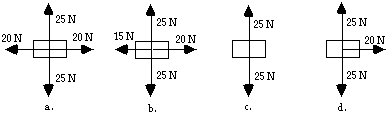If an object is moving at a constant speed in a constant rightward direction, then the acceleration is zero and the net force must be zero. Choice B and D show a rightward net force and therefore a rightward acceleration, inconsistent with the described motion.

 Useful Web Links The Big Misconception

[ #1 | #2 | #3 | #4 | #5 | #6 | #7 | #8 | #9 | #10 | #11 | #12 | #13 | #14 | #15 | #16 | #17 | #18 | #19 | #20 | #21 | #22 | #23 | #24 | #25 | #26 | #27 | #28 | #29 | #30 | #31 | #32 | #33 | #34 | #35 | #36 | #37 | #38 | #39 | #40 | #41 | #42 | #43 | #44 | #45 | #46 | #47 | #48 | #49 | #50 | #51 | #52 | #53 | #54 | #55 | #56 | #57 | #58 | #59 | #60 ]

13. According to Newton's third law, every force is accompanied by an equal and opposite reaction force. The reason that these forces do not cancel each other is ____.

a. the action force acts for a longer time period

b. the two forces are not always in the same direction

c. one of the two forces is greater than the other

d. the two forces act upon different objects; only forces on the same object can balance each other.

e. ... nonsense! They do cancel each other. Objects accelerate because of the presence of a third force.

Action and reaction forces always act upon the interacting objects for the same amount of time with the same magnitude. So if object A pushes on object B, then object B simultaneously pushes on object A with the same amount of force. The force on object B will be one of perhaps many forces which will govern its motion. But the reaction force is on object A and cannot contribute to object B's motion since it is not acting upon object B. Action-reaction forces can NEVER cancel each other.

 Useful Web Links Newton's Third Law || Action and Reaction Force Pairs

[ #1 | #2 | #3 | #4 | #5 | #6 | #7 | #8 | #9 | #10 | #11 | #12 | #13 | #14 | #15 | #16 | #17 | #18 | #19 | #20 | #21 | #22 | #23 | #24 | #25 | #26 | #27 | #28 | #29 | #30 | #31 | #32 | #33 | #34 | #35 | #36 | #37 | #38 | #39 | #40 | #41 | #42 | #43 | #44 | #45 | #46 | #47 | #48 | #49 | #50 | #51 | #52 | #53 | #54 | #55 | #56 | #57 | #58 | #59 | #60 ]

14. As you sit in your chair and study your physics (presuming that you do), the force of gravity acts downward upon your body. The reaction force to the force of the Earth pulling you downward is ___.

a. the force of the chair pushing you upward

b. the force of the floor pushing your chair upward

c. the force of the Earth pushing you upward

d. the force of air molecules pushing you upwards

e. the force of your body pulling the Earth upwards

f. ... nonsense! Gravity is a field force and there is no such reaction force.

The most common wrong answer is a - the force of the chair pushing you upward. As you sit in your chair, the chair is indeed pushing you upward but this is not the reaction force to the force of the Earth pulling you downward. The chair pushing you upward is the reaction force to you sitting on it and pushing the chair downward. To determine the action-reaction force pairs if given a statement of the form object A pulls X-ward on object B, simply take the subject and the object in the sentence and switch their places and then change the direction to the opposite direction (so the reaction force is object B pulls object A in the opposite direction of X). So if the Earth pulls you downward, then the reaction force is you pull the Earth upward.

 Useful Web Links Newton's Third Law || Action and Reaction Force Pairs

[ #1 | #2 | #3 | #4 | #5 | #6 | #7 | #8 | #9 | #10 | #11 | #12 | #13 | #14 | #15 | #16 | #17 | #18 | #19 | #20 | #21 | #22 | #23 | #24 | #25 | #26 | #27 | #28 | #29 | #30 | #31 | #32 | #33 | #34 | #35 | #36 | #37 | #38 | #39 | #40 | #41 | #42 | #43 | #44 | #45 | #46 | #47 | #48 | #49 | #50 | #51 | #52 | #53 | #54 | #55 | #56 | #57 | #58 | #59 | #60 ]

15. A golf pro places a ball at rest on the tee, lines up his shot, draws back his club, and lets one rip. During the contact of the golf club with the golf ball, the force of the club on the ball is ____ the force of the ball on the club and the acceleration of the club is ____ than the acceleration of the ball.

 a. greater than, greater than b. greater than, equal to c. greater than, less than d. less than, less than e. less than, equal to f. less than, greater than g. equal to, equal to h. equal to, greater than i. equal to, less than

For every action, there is an equal and opposite reaction force. In this case, the force on the club is equal to the force on the ball. The subsequent accelerations of the interacting objects will be inversely dependent upon mass. The more massive club will have less acceleration than the less massive ball.

 Useful Web Links Newton's Third Law || Action and Reaction Force Pairs || Newton's Second Law

[ #1 | #2 | #3 | #4 | #5 | #6 | #7 | #8 | #9 | #10 | #11 | #12 | #13 | #14 | #15 | #16 | #17 | #18 | #19 | #20 | #21 | #22 | #23 | #24 | #25 | #26 | #27 | #28 | #29 | #30 | #31 | #32 | #33 | #34 | #35 | #36 | #37 | #38 | #39 | #40 | #41 | #42 | #43 | #44 | #45 | #46 | #47 | #48 | #49 | #50 | #51 | #52 | #53 | #54 | #55 | #56 | #57 | #58 | #59 | #60 ]

Each one of Newton's Laws can play a role in any one particular situation. However, one of the laws is often most obviously dominant in governing the motion of a situation. Pick which of Newton's most governs the situations described below.

 a. First Law (inertia) b. Second Law (F = m•a) c. Third Law (action-reaction)

16. A helicopter must have two sets of blades in order to fly with stability.

17. If you were in an elevator and the cable broke, jumping up just before the elevator hit the ground would not save you. Sorry.

18. You usually jerk a paper towel from a roll in order to tear it instead of pulling it smoothly.

19. A student desk changes the amount of force it puts on other objects throughout a school day.

20. Heavy objects are not easier to move around in a horizontal fashion on the Moon than on the Earth.

21. The stronger, heavier team in a tug-of-war does not create a larger tension in the rope than the weaker, lighter team.

16. C - As the helicopter blades spin and push air in one direction, the air pushes the blades in the opposite direction; the result is that the helicopter can begin to rotate about the axis of the blade. To counteract this rotation, a second set of blades is required.

17. A - An object moving downwards will continue to move downwards unless acted upon by an unbalanced force. If you make an effort to supply such a force in an attempt to suddenly alter the direction of your motion, then you are creating a greater velocity change than if you merely hit the ground and stopped. If this greater velocity change occurred suddenly (in the same amount of time as the stopping of you and the elevator), then you would experience a greater acceleration, a greater net force, and a greater ouch mark than if you had merely hit the ground and stopped.

18. A - The paper towel is at rest and resists changes in its at rest state. So if you apply a sudden force to one of the paper towel sheets, the great mass of the remainder of the roll will resist a change in its at rest state and the roll will easily break at the perforation.

19. C - As a student sits in the seat, they are applying a downward force upon the seat. The reaction force is that the seat applies an upward force upon the person. A weightier person will apply more downward force than a lighter person. Thus, the seat will constantly be changing the amount of reaction force throughout the day as students of different weight sit in it.

20. A - All objects have inertia or a tendency to resist changes in their state of motion. This inertia is dependent solely upon mass and is subsequently not altered by changes in the gravitational environment. To move an object horizontally, one must apply a force; this force will be resisted by the mass or inertia of the object. On the moon, the object offers the same amount of inertia as on Earth; it is just as difficult (or easy) to move around.

21. C - A rope encounters tension when pulled on at both ends. The tension in the rope is everywhere the same. If team A were to pull at the left end, then the left end would pull back with the same amount of force upon team A. This force is the same everywhere in the rope, including at the end where team B is pulling. Thus team B is pulling back on the rope with the same force as team A. So if the forces are the same at each end, then how can a team ever win a tug-of-war. The way a stronger team wins a tug-of-war is with their legs. They push upon the ground with a greater force than the other team. This force upon the ground results in a force back upon the team in order for them to pull the rope and the other team backwards across the line.

 Useful Web Links Newton's First Law || Newton's Second Law || Newton's Third Law

[ #1 | #2 | #3 | #4 | #5 | #6 | #7 | #8 | #9 | #10 | #11 | #12 | #13 | #14 | #15 | #16 | #17 | #18 | #19 | #20 | #21 | #22 | #23 | #24 | #25 | #26 | #27 | #28 | #29 | #30 | #31 | #32 | #33 | #34 | #35 | #36 | #37 | #38 | #39 | #40 | #41 | #42 | #43 | #44 | #45 | #46 | #47 | #48 | #49 | #50 | #51 | #52 | #53 | #54 | #55 | #56 | #57 | #58 | #59 | #60 ]

For the next several questions, consider the velocity-time plot below for the motion of an object along a horizontal surface. The motion is divided into several time intervals, each labeled with a letter.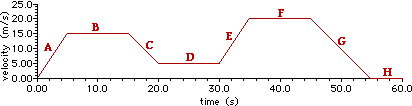22. During which time interval(s), if any, are there no forces acting upon the object? List all that apply.

23. During which time interval(s), if any, are the forces acting upon the object balanced? List all that apply.

24. During which time interval(s), if any, is there a net force acting upon the object? List all that apply.

25. During which time interval(s), if any, is the net force acting upon the object directed toward the right? List all that apply.

26. During which time interval(s), if any, is the net force acting upon the object directed toward the left? List all that apply.

22. None - If an object is on a surface, one can be guaranteed of at least two forces - gravity and normal force.

23. BDFH - If the forces are balanced, then an object is moving with a constant velocity. This is represented by a horizontal line on a velocity-time plot.

24. ACEG - If an object has a net force upon it, then it is accelerating. Acceleration is represented by a sloped line on a velocity-time plot.

25. AE - If the net force is directed to the right, then the acceleration is to the right (in the + direction). This is represented by a line with a + slope (i.e., upward slope).

26. CG - If the net force is directed to the left, then the acceleration is to the left (in the - direction). This is represented by a line with a - slope (i.e., downward slope).

 Useful Web Links The Meaning of Shape for a v-t Graph || Balanced and Unbalanced Forces || Newton's Second Law

[ #1 | #2 | #3 | #4 | #5 | #6 | #7 | #8 | #9 | #10 | #11 | #12 | #13 | #14 | #15 | #16 | #17 | #18 | #19 | #20 | #21 | #22 | #23 | #24 | #25 | #26 | #27 | #28 | #29 | #30 | #31 | #32 | #33 | #34 | #35 | #36 | #37 | #38 | #39 | #40 | #41 | #42 | #43 | #44 | #45 | #46 | #47 | #48 | #49 | #50 | #51 | #52 | #53 | #54 | #55 | #56 | #57 | #58 | #59 | #60 ]

For the next several questions, consider the dot diagram below for the motion of an object along a horizontal surface. The motion is divided into several time intervals, each labeled with a letter.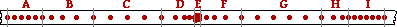27. During which time interval(s), if any, are there no forces acting upon the object? List all that apply.

28. During which time interval(s), if any, are the forces acting upon the object balanced? List all that apply.

29. During which time interval(s), if any, is there a net force acting upon the object? List all that apply.

30. During which time interval(s), if any, is the net force acting upon the object directed toward the right? List all that apply.

31. During which time interval(s), if any, is the net force acting upon the object directed toward the left? List all that apply.

27. None - If an object is on a surface, one can be guaranteed of at least two forces - gravity and normal force.

28. ACEGI - If the forces are balanced, then an object is moving with a constant velocity or at rest. This is represented by a section of a dot diagram where the dots are equally spaced apart (moving with a constant velocity) or not even spaced apart at all (at rest).

29. BDFH - If an object has a net force upon it, then it is accelerating. Acceleration is represented by a section of a dot diagram in which the spacing between consecutive dots is either increasing or decreasing.

30. BF - If the net force is directed to the right, then the acceleration is to the right (in the + direction). This is represented by a dot diagram in which the dots are increasing their separation distance as the object moves from left to right.

31. DH - If the net force is directed to the left, then the acceleration is to the left (in the - direction). This is represented by a dot diagram in which the dots are decreasing their separation distance as the object moves from left to right.

 Useful Web Links Ticker Tape Diagrams || Balanced and Unbalanced Forces || Newton's Second Law

[ #1 | #2 | #3 | #4 | #5 | #6 | #7 | #8 | #9 | #10 | #11 | #12 | #13 | #14 | #15 | #16 | #17 | #18 | #19 | #20 | #21 | #22 | #23 | #24 | #25 | #26 | #27 | #28 | #29 | #30 | #31 | #32 | #33 | #34 | #35 | #36 | #37 | #38 | #39 | #40 | #41 | #42 | #43 | #44 | #45 | #46 | #47 | #48 | #49 | #50 | #51 | #52 | #53 | #54 | #55 | #56 | #57 | #58 | #59 | #60 ]

For the next several questions, consider the trajectory diagram shown below for a projectile thrown at an angle to the horizontal. The vector arrows represent the horizontal and vertical components of the projectile's velocity. Several points in the trajectory are labeled with a letter. Use the trajectory diagram to answer the next several questions. (Consider air resistance to be negligible.)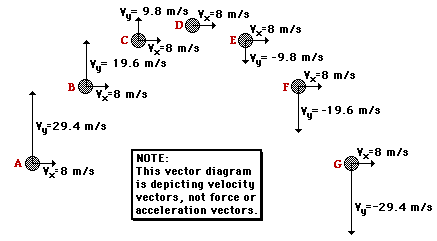32. At which point(s), if any, are there no forces acting upon the object? List all that apply.

33. At which point(s), if any, are the forces acting upon the object balanced? List all that apply.

34. At which point(s), if any, is there a net force acting upon the object? List all that apply.

35. At which point(s), if any, is the net force acting upon the object directed toward the right? List all that apply.

36. At which point(s), if any, is the net force acting upon the object directed upward? List all that apply.

32. None

33. None

34. ABCDEFG

35. None

36. None

This object is a projectile as can be seen by its constant horizontal velocity and a changing vertical velocity (besides that, the problem states that this is a projectile). A projectile is an object upon which the only force is gravity. Gravity acts downward to accelerate an object downward. This force is an unbalanced force or net force. It causes a vertical rising object to slow down and a falling object to speed up.

The presence of a horizontal velocity does not demand a horizontal force, only a balance of horizontal forces. Having no forces horizontally would cause the projectile to move at a constant horizontal speed once it is launched. Similarly an upward force is not needed on this projectile. When launched, an upward velocity is imparted to it; this velocity is steadily decreased as the object is acted upon by the downward force of gravity. An upward force would only be required for an object which is speeding up as it rises upward.

 Useful Web Links Projectile Trajectory || Balanced and Unbalanced Forces || Newton's Second Law

[ #1 | #2 | #3 | #4 | #5 | #6 | #7 | #8 | #9 | #10 | #11 | #12 | #13 | #14 | #15 | #16 | #17 | #18 | #19 | #20 | #21 | #22 | #23 | #24 | #25 | #26 | #27 | #28 | #29 | #30 | #31 | #32 | #33 | #34 | #35 | #36 | #37 | #38 | #39 | #40 | #41 | #42 | #43 | #44 | #45 | #46 | #47 | #48 | #49 | #50 | #51 | #52 | #53 | #54 | #55 | #56 | #57 | #58 | #59 | #60 ]

## Part D: Drawing and Interpreting Free-Body Diagrams

37. Construct free-body diagrams for the following physical situations at the instant in time for which they are described. As is always done in free-body diagrams, label the forces according to type and draw the arrows such that their length reflects the magnitude of the force.

 a. A book is at rest on top of a table.b. A book is being pushed to the right across a table surface with a constant velocity. (Neglect Fair.)c. A book is being pushed to the right across a table surface and accelerating in the direction of the push. (Neglect Fair.)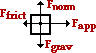d. A student is pushing lightly upon a large box in an attempt to push it to the right across the floor, but the box fails to move.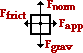e. A rightward-moving box (which was previously set into rightward motion across the floor) gradually slows to a stop.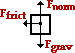f. An air track glider is gliding to the right at constant velocity.(or replace the Fnorm with Fair) g. A ball is dropped from rest from the top of a building. (Neglect Fair.)h. Several seconds after being thrown, a football is moving upwards and rightwards towards the peak of its trajectory. (Neglect Fair.)i. Several seconds after being thrown, a football reaches the precise peak of its trajectory. (Neglect Fair.)j. A falling skydiver is speeding up.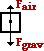k. A falling skydiver has reached a terminal velocity.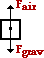l. After reaching a terminal velocity, a falling skydiver has opened her parachute.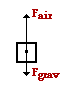m. A car is moving to the right at a high speed across a level roadway surface; the driver's foot remains on the gas pedal.n. A car is skidding to a stop (with wheels locked) while traveling to the right across a level roadway surface.(Fair could be added to Ffrict) o. An elevator (held by a cable) is moving upwards at a constant velocity. (Neglect Fair.)p. An upward moving elevator (held by a cable) slows down. (Neglect Fair.)q. A downward moving elevator (held by a cable) slows down. (Neglect Fair.)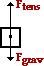r. A picture hangs symmetrically by two wires oriented at angles to the vertical.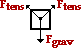Useful Web Links Types of Forces || Drawing Free-Body Diagrams

[ #1 | #2 | #3 | #4 | #5 | #6 | #7 | #8 | #9 | #10 | #11 | #12 | #13 | #14 | #15 | #16 | #17 | #18 | #19 | #20 | #21 | #22 | #23 | #24 | #25 | #26 | #27 | #28 | #29 | #30 | #31 | #32 | #33 | #34 | #35 | #36 | #37 | #38 | #39 | #40 | #41 | #42 | #43 | #44 | #45 | #46 | #47 | #48 | #49 | #50 | #51 | #52 | #53 | #54 | #55 | #56 | #57 | #58 | #59 | #60 ]

## Part E: Force-Mass-Acceleration Relationships

Construct free-body diagrams for the following objects; label the forces according to type. Use the approximation that g=10 m/s2
to determine the magnitude of all forces and the net force and acceleration of the object.

 38. A 2-kg box is at rest on a table.("At rest" would indicate a balance of forces and an acceleration of 0 m/s/s.) 39. A 2-kg box is free-falling from the table to the ground.("Free-falling" indicates that the only force that influences the motion is the force of gravity.) 40. A 2-kg box equipped with a parachute is falling at a terminal velocity after being dropped from a plane.(A "terminal velocity" indicates a constant velocity and a balance of forces.)

[ #1 | #2 | #3 | #4 | #5 | #6 | #7 | #8 | #9 | #10 | #11 | #12 | #13 | #14 | #15 | #16 | #17 | #18 | #19 | #20 | #21 | #22 | #23 | #24 | #25 | #26 | #27 | #28 | #29 | #30 | #31 | #32 | #33 | #34 | #35 | #36 | #37 | #38 | #39 | #40 | #41 | #42 | #43 | #44 | #45 | #46 | #47 | #48 | #49 | #50 | #51 | #52 | #53 | #54 | #55 | #56 | #57 | #58 | #59 | #60 ]

 41. A 2-kg box is sliding to the right across a table. The force of friction upon the box is 5 N.(Friction is directed opposite the motion and causes a leftward acceleration; no rightward force is spoken of, only a rightward motion.) 42. An 8-N force is applied to a 2-kg box to move it to the right across the table at a constant velocity of 1.5 m/s.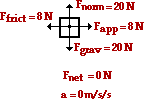(A "constant velocity" indicates an acceleration of 0 m/s/s and a balance of forces.) 43. An 8-N force is applied to a 2-kg box to accelerate it to the right across a table. The box encounters a force of friction of 5 N.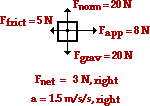(The horizontal forces can be summed as vectors; divide by the mass to obtain the acceleration value.) 44. A 500-kg freight elevator is descending down through the shaft at a constant velocity of 0.50 m/s.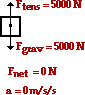(A "constant velocity" indicates an acceleration of 0 m/s/s and a balance of forces.) 45. A 500-kg freight elevator is moving upwards towards its destination. Near the end of the ascent, the upward moving elevator encounters a downward acceleration of 2.0 m/s/s.(Begin by multiplying m•a to find the net force - 1000 N, down. The downward gravity force must be 1000 N more than the upward tension force.) 46. A 150-N rightward force is applied to a 20-kg box to accelerate it to the right across a rough surface at a rate of 2.0 m/s/s.(Begin by multiplying m•a to determine the net force - 40 N, right. The rightward applied force must be 40 N more than the leftward friction force.)

 Useful Web Links Free-Body Diagrams || Finding Acceleration || Finding Individual Forces

[ #1 | #2 | #3 | #4 | #5 | #6 | #7 | #8 | #9 | #10 | #11 | #12 | #13 | #14 | #15 | #16 | #17 | #18 | #19 | #20 | #21 | #22 | #23 | #24 | #25 | #26 | #27 | #28 | #29 | #30 | #31 | #32 | #33 | #34 | #35 | #36 | #37 | #38 | #39 | #40 | #41 | #42 | #43 | #44 | #45 | #46 | #47 | #48 | #49 | #50 | #51 | #52 | #53 | #54 | #55 | #56 | #57 | #58 | #59 | #60 ]

## Part F: Problem Solving

For the following problems, draw free-body diagrams and solve for the requested unknown. Use g = 9.8 m/s/s.

 Useful Web Links Free-Body Diagrams || Finding Acceleration || Finding Individual Forces || Kinematic Equations and Problem-Solving

47. A 0.250-kg ball is thrown upwards with an initial velocity of 12.0 m/s at an angle of 30.0 degrees. Determine the acceleration of the ball when it has reached the peak of its trajectory. Assume negligible air resistance. PSYWThere is only one force upon the ball - the force of gravity. (Air resistance is negligible; the ball is not on a surface, so there is no friction or normal force; the applied force which projects it into motion does not act upon the ball during its trajectory; there are no springs, strings, wires, or cables attached so there is neither a tension force nor a spring force.) The force of gravity acts downward with a magnitude of m•g = (0.250 kg) •(9.8 m/s/s) = 2.45 N. The net force is 2.45 N; when divided by mass, the acceleration can be found.

a = Fnet / m = (2.45 N, down) / (0.250 kg) = 9.8 m/s/s, down

48. A 72-kg skydiver is falling from 10000 feet. At an instant during the fall, the skydiver encounters an air resistance force of 540 Newtons. Determine the acceleration of the skydiver at this instant. PSYWThere are two forces acting upon the skydiver - gravity (down) and air resistance (up).  The force of gravity has a magnitude of m•g = (72 kg) •(9.8 m/s/s) = 706 N. The sum of the vertical forces is

Fy = 540 N, up + 706 N, down = 166 N, down

The acceleration of the skydiver can be computed using the equationFy = m•ay.

ay = (166 N, down) / (72 kg) = 2.3 m/s/s, down

49. A 72-kg skydiver is falling from 10 000 feet. After reaching terminal velocity, the skydiver opens his parachute. Shortly thereafter, there is an an instant in time in which the skydiver encounters an air resistance force of 1180 Newtons. Determine the acceleration of the skydiver at this instant. PSYWThere are two forces acting upon the skydiver - gravity (down) and air resistance (up). The force of gravity has a magnitude of m•g = (72 kg) •(9.8 m/s/s) = 706 N. The sum of the vertical forces is

Fy = 1180 N, up + 706 N, down = 474 N, up

The acceleration of the skydiver can be computed using the equation Fy = m•ay.

ay = (474 N, up) / (72 kg) = 6.6 m/s/s, up

50. A 5.20-N force is applied to a 1.05-kg object to accelerate it rightwards across a friction-free surface. Determine the acceleration of the object. (Neglect air resistance.) PSYWUpon neglecting air resistance, there are three forces acting upon the object. The up and down force balance each other and the acceleration is caused by the applied force. The net force is 5.20 N, right (equal to the only rightward force - the applied force). So the acceleration of the object can be computed using Newton's second law.

a = Fnet / m = (5.20 N, right) / (1.05 kg) = 4.95 m/s/s, right

51. A 5.20-N force is applied to a 1.05-kg object to accelerate it rightwards. The object encounters 3.29-N of friction. Determine the acceleration of the object. (Neglect air resistance.) PSYWUpon neglecting air resistance, there are four forces acting upon the object. The up and down forces balance each other. The acceleration is rightward since the rightward applied force is greater than the leftward friction force. The horizontal forces can be summed as vectors in order to determine the net force.

Fnet = ∑Fx = 5.20 N, right - 3.29 N, left = 1.91 N, right

The acceleration of the object can be computed using Newton's second law.

ax∑Fx / m = (1.91 N, down) / (1.05 kg) = 1.82 m/s/s, right

52. Determine the applied force required to accelerate a 3.25-kg object rightward with a constant acceleration of 1.20 m/s/s if the force of friction opposing the motion is 18.2 N. (Neglect air resistance.) PSYWThere are four forces acting upon the object as shown in the free-body diagram at the right. The two vertical forces must balance since there is no vertical acceleration. Since the mass and the acceleration are known, the net force can be computed:

Fnet = m•a = (3.25 kg, right) • (1.20 m/s/s) = 3.90 N, right

Since the acceleration is to the right, the net force is directed to the right. Thus, the rightward force (applied force) must exceed the leftward force (friction force) by an amount equal to the 3.9 N. So the applied force can be computed.

Fapp = Ffrict + Fnet = 18.2 N + 3.90 N = 22.1 N

53. A 921-kg sports car is moving rightward with a speed of 29.0 m/s. The driver suddenly slams on the brakes and the car skids to a stop over the course of 3.20 seconds with the wheels locked. Determine the average resistive force acting upon the car. PSYWThere are three (perhaps four) forces acting upon this car. There is the upward force (normal force) and the downward force (gravity); these two forces balance each other since there is no vertical acceleration. The resistive force is likely a combination of friction and air resistance. These forces act leftward upon a rightward skidding car. In the free-body diagram, these two forces are represented by the Ffrict arrow.

The acceleration is not given but can be calculated from the kinematic information that is given:

vi = 29.0 m/s, vf = 0 m/s, and t = 3.20 s

The acceleration of the object is the velocity change per time:

a = Delta v / t = (0 m/s - 29.0 m/s) / (3.20 s) = -9.67 m/s/s or 9.67 m/s/s, left.

This acceleration can be used to determine the net force:

Fnet = m•a = (921 kg) • (9.67 m/s/s, left) = 8350 N, left

The friction forces (surface and air) provide this net force and are equal in magnitude to this net force.

54. A 0.250-kg rightward moving air track glider decreases its speed from 0.872 m/s to 0.798 m/s over the length of a 1.71-m long air track. Determine the average resistive force acting upon the air track glider. PSYWThis problem is similar to the previous problem. There are three (and perhaps four) forces acting upon the glider. The up force could be considered to be a normal force or an air resistance force (from the air blowing through the air track holes). Like the previous problem, the acceleration is not given but it can be calculated from the kinematic information:

vi = 0.872 m/s, vf = 0.798 m/s, and d = 1.71 m

Using a kinematic equation, the values for initial and final velocity and displacement can be substituted; the algebraic steps are shown below.

vf2 = vi2 + 2•a•d

(0.872 m/s)2 = (0.798 m/s)2 + 2•a•(1.71 m)

(0.872 m/s)2 - (0.798 m/s)2 = 2•a•(1.71 m)

0.124 m2/s2 = (3.42 m)•a

0.0361 m/s/s = a

This acceleration can be used to determine the net force:

Fnet = m•a = (0.250 kg) • (0.0361 m/s/s, left) = 0.00903 N, left

The friction forces (surface and air) provide this net force and are equal in magnitude to this net force.

55. A 1250-kg small aircraft decelerates along the runway from 36.6 m/s to 6.80 m/s in 5.10 seconds. Determine the average resistive force acting upon the plane. (Assume that its engine/propeller makes no contributes to its forward motion). PSYWThis problem is similar to the two previous problems in many respects: the free-body diagram is identical or similar and the acceleration is not given but determinable from the kinematic information.

vi = 36.6 m/s, vf = 6.80 m/s, and t = 5.10 s

The acceleration of the object is the velocity change per time:

a = Delta v / t = (6.80 m/s - 36.6 m/s) / (5.10 s) = -5.84 m/s/s or 5.84 m/s/s, left.

This acceleration can be used to determine the net force:

Fnet = m•a = (1250 kg) • (5.84 m/s/s, left) = 7.30 x 103 N, left

The friction forces (surface and air) provide this net force and are equal in magnitude to this net force.

56. A tow truck exerts a 18300-N force upon a 1210 car to drag it out of a mud puddle onto the shoulder of a road. A 17900 N force opposes the car's motion. The plane of motion of the car is horizontal. Determine the time required to drag the car a distance of 6.90 meters from its rest position. PSYWUpon neglecting air resistance, there are four forces acting upon the object. The up and down forces balance each other. The acceleration is rightward (or in the direction of the applied force) since the rightward applied force is greater than the leftward friction force. The horizontal forces can be summed as vectors in order to determine the net force.

Fnet = ·Fx = 18300 N, right - 17900 N, left = 400 N, right

The acceleration of the object can be computed using Newton's second law.

ax = ·Fx / m = (400 N, down) / (1210 kg) = 0.3306 m/s/s, right

This acceleration value can be combined with other known kinematic information (vi = 0 m/s, d = 6.90 m) to determine the time required to drag the car a distance of 6.9 m. The following kinematic equation is used; substitution and algebra steps are shown.

d = vi • t + 0.5 •a • t2

d = vi • t + 0.5 •a • t2

6.90 m = 0.5 • (0.3306 m/s/s) • t2

6.90 m / (0.5 • 0.3306 m/s/s ) = t2

41.4 = t2

6.46 s = t

57. A 4.44-kg bucket suspended by a rope is accelerated upwards from an initial rest position. If the tension in the rope is a constant value of 83.1 Newtons, then determine the speed (in m/s) of the bucket after 1.59 seconds. PSYWThere are two forces acting upon the bucket - the force of gravity (up) and the tension force (down). The magnitude of the force of gravity is found from m•g; its value is 43.5 N. These two forces can be summed as vectors to determine the net force.

Fnet = ·Fy = 83.1 N, up + 43.5 N, down = 39.6 N, up

The acceleration can be calculated using Newton's second law of motion.

a = Fnet / m = (39.6 N, up) / (4.44 kg) = 8.92 m/s/s, up

The acceleration value can be used with other kinematic information (vi = 0 m/s, t = 1.59 s) to calculate the final speed of the bucket. The kinematic equation, substitution and algebra steps are shown.

vf = vi + a•t

vf = 0 m/s + (8.92 m/s/s)•(1.59 s)

vf = 14.2 m/s

58. A 22.6-N horizontal force is applied to a 0.0710-kg hockey puck to accelerate it across the ice from an initial rest position. Ignore friction and determine the final speed (in m/s) of the puck after being pushed for a time of .0721 seconds. PSYWUpon neglecting air resistance, there are three forces acting upon the object. The up and down force balance each other and the acceleration is caused by the applied force. The net force is 22.6 N, right (equal to the only rightward force - the applied force). So the acceleration of the object can be computed using Newton's second law.

a = Fnet / m = (22.6 N, right) / (0.0710 kg) = 318 m/s/s, right

The acceleration value can be used with other kinematic information (vi = 0 m/s, t = 0.0721 s) to calculate the final speed of the puck. The kinematic equation, substitution and algebra steps are shown.

vf = vi + a•t

vf = 0 m/s + (318 m/s/s)•(0.0721 s)

vf = 23.0 m/s

59. A train has a mass of 6.32x104 kg and is moving with a speed of 94.3 km/hr. The engineer applies the brakes which results in a net backward force of 2.43x105 Newtons on the train. The brakes are held for 3.40 seconds. How far (in meters) does the train travel during this time? PSYWThere are three (perhaps four) forces acting upon this train. There is the upward force (normal force) and the downward force (gravity); these two forces balance each other since there is no vertical acceleration. The resistive force is likely a combination of friction and air resistance. These forces act leftward upon a rightward skidding train. In the free-body diagram, these two forces are represented by the Ffrict arrow. The value of this resistive force is given as 2.43x105 N. This is the net force since there are no other horizontal forces; it is the force which causes the acceleration of the train. The acceleration value can be determined using Newton's second law of motion.

a = Fnet / m = (2.43x105 N) / (6.32x104 kg) = 3.84 m/s/s, left

This acceleration value can be combined with other kinematic variables (vi = 94.3 km/hr = 26.2 m/s; t = 3.40 s) in order to determine the distance the train travels in 3.4 seconds.

d = vi • t + 0.5 •a • t2

d = (26.2 m/s) • (3.40 s) + 0.5 • (-3.84 m/s/s) • (3.40 s)2

d = 89.1 m - 22.2 m

d = 66.8 m

60. A shopper in a supermarket pushes a loaded cart with a horizontal force of 16.5 Newtons. If the cart has a mass of 33.8 kg, how far (in meters) will it move in 1.31 seconds, starting from rest? (Neglect resistive forces.) PSYWUpon neglecting air resistance, there are three forces acting upon the object. The up and down force balance each other and the acceleration is caused by the applied force. The net force is 22.6 N, right (equal to the only rightward force - the applied force). So the acceleration of the object can be computed using Newton's second law.

a = Fnet / m = (16.5 N, right) / (33.8 kg) = 0.488 m/s/s, right

The acceleration value can be used with other kinematic information (vi = 0 m/s, t = 1.31 s) to calculate the final speed of the cart. The kinematic equation, substitution and algebra steps are shown.

d = vi • t + 0.5 •a • t2

d = vi • t + + 0.5 • (0.488 m/s/s)•(1.31 s) 2

d = 0.419 m

 Useful Web Links Free-Body Diagrams || Finding Acceleration || Finding Individual Forces || Kinematic Equations and Problem-Solving

[ #1 | #2 | #3 | #4 | #5 | #6 | #7 | #8 | #9 | #10 | #11 | #12 | #13 | #14 | #15 | #16 | #17 | #18 | #19 | #20 | #21 | #22 | #23 | #24 | #25 | #26 | #27 | #28 | #29 | #30 | #31 | #32 | #33 | #34 | #35 | #36 | #37 | #38 | #39 | #40 | #41 | #42 | #43 | #44 | #45 | #46 | #47 | #48 | #49 | #50 | #51 | #52 | #53 | #54 | #55 | #56 | #57 | #58 | #59 | #60 ]

## Navigate to:

Review Session Home - Topic Listing

Newton's Laws - Home  ||  Printable Version  ||  Questions with Links

Answers to Questions:  All  ||  #1-7  ||  #8-36  ||  #37-46  ||  #47-60

## You Might Also Like ...

Users of The Review Session are often looking for learning resources that provide them with practice and review opportunities that include built-in feedback and instruction. If that is what you're looking for, then you might also like the following:

The Calculator Pad includes physics word problems organized by topic. Each problem is accompanied by a pop-up answer and an audio file that explains the details of how to approach and solve the problem. It's a perfect resource for those wishing to improve their problem-solving skills.

2. Minds On Physics the App Series

Minds On Physics the App ("MOP the App") is a series of interactive questioning modules for the student that is serious about improving their conceptual understanding of physics. Each module of the series covers a different topic and is further broken down into sub-topics. A "MOP experience" will provide a learner with challenging questions, feedback, and question-specific help in the context of a game-like environment. It is available for phones, tablets, Chromebooks, and Macintosh computers. It's a perfect resource for those wishing to refine their conceptual reasoning abilities. Part 2 of the series includes Newton's Laws of Motion.

Visit: MOP the App Home || MOP the App - Part 2